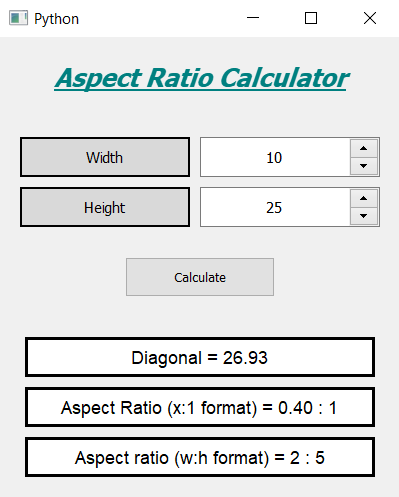Open in App
Not now

# Aspect Ratio Calculator using PyQt5

• Difficulty Level : Hard
• Last Updated : 12 Sep, 2022

In this article we will see how we can create a aspect ratio calculator using PyQt5. The aspect ratio of an image is the ratio of its width to its height. It is commonly expressed as two numbers separated by a colon, as in 16:9. For an x:y aspect ratio, the image is x units wide and y units high. Below is how the calculator will lookPyQt5 is cross-platform GUI toolkit, a set of python bindings for Qt v5. One can develop an interactive desktop application with so much ease because of the tools and simplicity provided by this library. Below is the command to install the PyQt5

`pip install PyQt5`

Concept:
User has to select a width and height for which calculator will find the diagonal and aspect ratio values, below is the formula for calculating diagonal

`diagonal = math.sqrt(width**2 + height**2)`

Below is the formula for calculating aspect Ratio (x:1 format)

`x = width / height`

Below is the formula for calculating aspect Ratio (width:height format)

```w = width / gcd
h = height / gcd```

Here gcd is the Highest Common Factor (HCF) of width and height

GUI Implementation Steps :
1. Create a heading label that display the calculator name
2. Create two labels for telling user to enter width and height
3. Create two spin box for entering width and height
4. Create a push button for calculating the ratios
5. Create three result labels for showing three various results

Back-End Implementation :
1. Set range to each of the spin box with minimum value equal to 1 so that user can’t enter 0 as input
2. Set various properties like alignment, geometry to each of the widget in the window
3. Add action to the push button when it get clicked
4. Inside the push button action get the width and height from the spin boxes
5. Calculate the diagonal value and do formatting of it and show the diagonal value with the help of first result label
6. Calculate the aspect ratio by diving each other this will prove aspect ratio in x : 1 format
7. Show this ratio with the help of second result label
8. Find the gcd of width and height with the help of Euclidean Algorithm
9. Divide the width and height with the gcd
10. Show the new width and height in aspect ratio format with the help of third label

Below is the implementation

## Python3

 `# importing libraries``from` `PyQt5.QtWidgets ``import` `*` `from` `PyQt5 ``import` `QtCore, QtGui``from` `PyQt5.QtGui ``import` `*` `from` `PyQt5.QtCore ``import` `*` `import` `math` `import` `sys`  `class` `Window(QMainWindow):` `    ``def` `__init__(``self``):``        ``super``().__init__()` `        ``# setting title``        ``self``.setWindowTitle(``"Python "``)` `        ``# width of window``        ``self``.w_width ``=` `400` `        ``# height of window``        ``self``.w_height ``=` `460` `        ``# setting geometry``        ``self``.setGeometry(``100``, ``100``, ``self``.w_width, ``self``.w_height)` `        ``# calling method``        ``self``.UiComponents()` `        ``# showing all the widgets``        ``self``.show()` `    ``# method for components``    ``def` `UiComponents(``self``):` `        ``# creating head label``        ``head ``=` `QLabel(``"Aspect Ratio Calculator"``, ``self``)` `        ``# setting geometry to the head``        ``head.setGeometry(``0``, ``10``, ``400``, ``60``)` `        ``# font``        ``font ``=` `QFont(``'Times'``, ``15``)``        ``font.setBold(``True``)``        ``font.setItalic(``True``)``        ``font.setUnderline(``True``)` `        ``# setting font to the head``        ``head.setFont(font)` `        ``# setting alignment of the head``        ``head.setAlignment(Qt.AlignCenter)` `        ``# setting color effect to the head``        ``color ``=` `QGraphicsColorizeEffect(``self``)``        ``color.setColor(Qt.darkCyan)``        ``head.setGraphicsEffect(color)` `        ``# creating a label``        ``w_label ``=` `QLabel(``"Width"``, ``self``)` `        ``# setting properties to the  label``        ``w_label.setAlignment(Qt.AlignCenter)``        ``w_label.setGeometry(``20``, ``100``, ``170``, ``40``)``        ``w_label.setStyleSheet(``"QLabel"``                              ``"{"``                              ``"border : 2px solid black;"``                              ``"background : rgba(70, 70, 70, 35);"``                              ``"}"``)``        ``w_label.setFont(QFont(``'Times'``, ``9``))` `        ``# creating a spin box``        ``self``.w_spin ``=` `QSpinBox(``self``)` `        ``# setting geometry to the spin box``        ``self``.w_spin.setGeometry(``200``, ``100``, ``180``, ``40``)` `        ``# setting range to the spin box``        ``self``.w_spin.setRange(``1``, ``999999``)` `        ``# setting font and alignment``        ``self``.w_spin.setFont(QFont(``'Times'``, ``9``))``        ``self``.w_spin.setAlignment(Qt.AlignCenter)` `        ``# creating a label``        ``h_label ``=` `QLabel(``"Height"``, ``self``)` `        ``# setting properties to the label``        ``h_label.setAlignment(Qt.AlignCenter)``        ``h_label.setGeometry(``20``, ``150``, ``170``, ``40``)``        ``h_label.setStyleSheet(``"QLabel"``                              ``"{"``                              ``"border : 2px solid black;"``                              ``"background : rgba(70, 70, 70, 35);"``                              ``"}"``)``        ``h_label.setFont(QFont(``'Times'``, ``9``))` `        ``# creating a spin box``        ``self``.h_spin ``=` `QSpinBox(``self``)` `        ``# setting geometry to the spin box``        ``self``.h_spin.setGeometry(``200``, ``150``, ``180``, ``40``)` `        ``# setting range``        ``self``.h_spin.setRange(``1``, ``999999``)` `        ``# setting font and alignment``        ``self``.h_spin.setFont(QFont(``'Times'``, ``9``))``        ``self``.h_spin.setAlignment(Qt.AlignCenter)` `        ``# creating a push button``        ``calculate ``=` `QPushButton(``"Calculate"``, ``self``)` `        ``# setting geometry to the push button``        ``calculate.setGeometry(``125``, ``220``, ``150``, ``40``)` `        ``# adding action to the calculate button``        ``calculate.clicked.connect(``self``.calculate_action)` `        ``# creating a label``        ``self``.result1 ``=` `QLabel(``self``)` `        ``# setting properties to result label``        ``self``.result1.setAlignment(Qt.AlignCenter)``        ``self``.result1.setGeometry(``25``, ``300``, ``350``, ``40``)``        ``self``.result1.setStyleSheet(``"QLabel"``                                  ``"{"``                                  ``"border : 3px solid black;"``                                  ``"background : white;"``                                  ``"}"``)``        ``self``.result1.setFont(QFont(``'Arial'``, ``11``))` `        ``# creating a label``        ``self``.result2 ``=` `QLabel(``self``)` `        ``# setting properties to result label``        ``self``.result2.setAlignment(Qt.AlignCenter)``        ``self``.result2.setGeometry(``25``, ``350``, ``350``, ``40``)``        ``self``.result2.setStyleSheet(``"QLabel"``                                   ``"{"``                                   ``"border : 3px solid black;"``                                   ``"background : white;"``                                   ``"}"``)``        ``self``.result2.setFont(QFont(``'Arial'``, ``11``))` `        ``# creating a label``        ``self``.result3 ``=` `QLabel(``self``)` `        ``# setting properties to result label``        ``self``.result3.setAlignment(Qt.AlignCenter)``        ``self``.result3.setGeometry(``25``, ``400``, ``350``, ``40``)``        ``self``.result3.setStyleSheet(``"QLabel"``                                   ``"{"``                                   ``"border : 3px solid black;"``                                   ``"background : white;"``                                   ``"}"``)``        ``self``.result3.setFont(QFont(``'Arial'``, ``11``))` `    ``def` `calculate_action(``self``):` `        ``# getting width``        ``width ``=` `self``.w_spin.value()` `        ``# getting height``        ``height ``=` `self``.h_spin.value()` `        ``# calculating diagonal``        ``diagonal ``=` `width``*``*``2` `+` `height``*``*``2``        ``diagonal ``=` `math.sqrt(diagonal)` `        ``# doing formatting of diagonal``        ``diagonal ``=` `'%.2f'` `%` `diagonal` `        ``# setting text to the result 1``        ``self``.result1.setText(``"Diagonal = "` `+` `diagonal)` `        ``# calculating aspect ratio (x:1 format)``        ``x ``=` `width ``/` `height` `        ``# formatting X value``        ``x ``=` `'%.2f'` `%` `x` `        ``# setting text to the result 2``        ``self``.result2.setText(``"Aspect Ratio (x:1 format) = "` `+` `x ``+` `" : 1"``)` `        ``# calculating aspect ratio (w:h format)``        ``# method to calculate GCD using Euclidean Algorithm``        ``def` `computeGCD(x, y):``            ``# looping``            ``while` `(y):``                ``x, y ``=` `y, x ``%` `y` `            ``# returning gcd value``            ``return` `x` `        ``gcd ``=` `computeGCD(width, height)` `        ``# setting width and height value``        ``width ``=` `width``/``/``gcd``        ``height ``=` `height``/``/``gcd` `        ``# setting text to the result 3``        ``self``.result3.setText(``"Aspect ratio (w:h format) = "` `+` `str``(width) ``+` `" : "` `+` `str``(height))`  `# create pyqt5 app``App ``=` `QApplication(sys.argv)` `# create the instance of our Window``window ``=` `Window()` `# start the app``sys.exit(App.``exec``())`

Output :

My Personal Notes arrow_drop_up